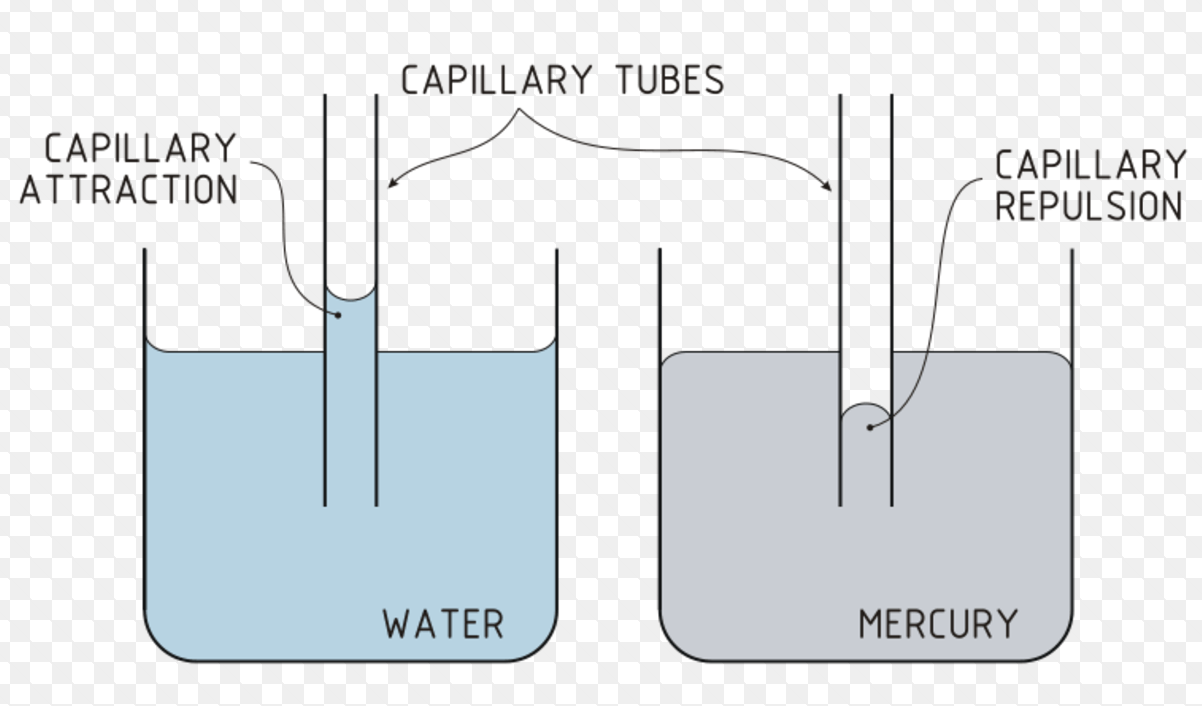> > Surface Tension

# Surface Tension

Have you ever noticed that people heat glass to round off the sharp edges? Why do they do that? Why doesn’t the glass break or melt? This is the concept of surface tension. In this chapter, we will look at this concept in greater depth. We will also discuss a few practical applications of the surface tension.

### Suggested VideosAtmospheric PressureIntroduction to FlotationVST Fluid Mechanics Problem 2 and its Solution## Surface Tension

Surface tension is the property of liquid which arises due to the fact that the molecules of the liquid at the surface are in a different situation than those in the interior of the liquid. A molecule lying inside the liquid is surrounded by other molecules and so is attracted equally in all directions. Hence, the net force of attraction acting on the molecule is zero.

A molecule lying on the surface is attracted more by the molecules lying in the bulk of the liquid than by the molecules lying above it in the vapour phase. A molecule lying on the surface experiences a net inward attraction. As a result of this inward pull on all molecules lying on the surface, the surface behaves as if it were under tension.In other words, the surface tension of a liquid is defined as the force acting at right angles to the surface along 1 cm length of the surface. Thus, the units of surface tension are dynes per centimetre or Newton per metre i.e. Nm-1 in the S.I. system.

As a result of inward pull on the molecules at the surface, the surface of the liquid tends to the smallest possible area for a given volume of the liquid. This gives the lowest energy state of the liquid. The drop of a liquid is spherical because, for a given volume, a sphere has minimum surface area.

The work in ergs required to be done to increase or extend the surface area by 1 sq cm is called surface energy. The units of surface energy are, therefore, ergs per sq cm or joules per sq m in S.I. system.

Surface Energy = work per sq cm= (Force× length) per sq cm = dynes cm-1

### The Spherical Shape of Droplets

The lowest energy state of a liquid will be when the surface area is minimum. Surface tension tries to decrease the surface area of the liquid to the minimum. The drops of a liquid are spherical because, for a given volume, a sphere has minimum surface area.

### Fire Polishing of Glass

Sharp glass edges are heated to make them smooth. This is because, on heating, the glass melts and takes up rounded shape at the edges which have minimum surface area. This is called fire polishing of glass.

### The Rise of Liquids in Capillary Tubes

If one end of a capillary tube is put into a liquid that wets glass, it is found that the liquid rises into the capillary tube to a certain height. This rise is due to the inward pull of surface tension acting on the surface which pushes the liquid into the capillary tube. It is because of the same reason that oil rises into the Wick of an oil lamp or water below the surface of the earth rises in the plant or ink in a blotting paper.

In case of liquids which do not wet glass, like Mercury, the level inside the capillary falls below the level outside, whereas the upper surface of a liquid that wets glass is concave, that of mercury is convex.A meniscus is a curved surface of a liquid. Cohesive forces are the attractive forces existing between the molecules of the same substance. For example, the cohesive force exists between the molecules of water or molecules of Mercury.

Adhesive forces are the attractive forces existing between the different substances. For example, adhesive forces exist between water and glass or Mercury and glass. In case of water taken in a glass tube, adhesive forces are stronger than Cohesive forces whereas it is reverse for Mercury taken in a glass tube.

## Solved Examples for You

Q: Describe the effect of nature of liquid and temperature on surface tension.

SolutionSurface tension is a property that arises due to the intermolecular forces of attraction among the molecules of the liquid. Greater are the intermolecular forces of attraction, higher is the surface tension of that liquid. Now, let us explain the effect of temperature on surface tension.

The surface tension of liquid generally decreases with the increase in temperature and becomes zero at the critical temperature. The decrease in surface tension with an increase of temperature is due to the fact that with an increase in temperature, the kinetic energy of the molecules increases. Therefore, the intermolecular attraction decreases.

Q: The radii of the two columns is U-tube are r1 and r2. When a liquid of density ρ (angle of contact is 0o) is filled in it, the level difference of liquid in two arms is h. Find out the surface tension of the liquid.

Solution: We know that, h = 2T/rρg
So,  h1 = 2T/r1ρg
h2 = 2T/r2ρg
h1– h2 = h = 2T/ρg(1/r1 – 1/r2)
Thus, T = hρgr1r2/2(r– r1)
If the respective values are known, an exact value of surface tension can be obtained.

Share with friends

## Customize your course in 30 seconds

##### Which class are you in?
5th
6th
7th
8th
9th
10th
11th
12th
Get ready for all-new Live Classes!
Now learn Live with India's best teachers. Join courses with the best schedule and enjoy fun and interactive classes.Ashhar Firdausi
IIT Roorkee
BiologyDr. Nazma Shaik
VTU
ChemistryGaurav Tiwari
APJAKTU
Physics
Get Started

## Browse

##### Mechanical Properties of FluidsSubscribe
Notify of

## Question Mark?

Have a doubt at 3 am? Our experts are available 24x7. Connect with a tutor instantly and get your concepts cleared in less than 3 steps.